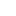# Category: Tutorial

## Basic Electronics Introduction Semiconductor material

In these tutorials of basic electronics, we will learn topics like BJT and MOSFET. They are the field-effect transistors and also the operational amplifiers in a short opamp, so first, we will complete the semiconductor...

## Digital Electronics Tutorial Basic Introduction

In this series of digital electronics, also known as digital logic or digital logic and designing. In this tutorial of digital electronics, we will first see what a signal is, then number systems and codes under that we are...

## Difference between BJT and FET

BJT and FET are electronic devices, and both are the family of transistors. Both transistors have the property of conduction as well as insulation.BJT, as well as FET, consists of three primary terminals in it. The basic...

## Number System

What is the number system? The number system can be defined as the language of a number.In digital electronics, the number system used for representing the information. In digital electronics the number system are two types:...